Courses

# Long Answer Type Questions- Surface Areas and Volumes Class 10 Notes | EduRev

## Class 10 : Long Answer Type Questions- Surface Areas and Volumes Class 10 Notes | EduRev

The document Long Answer Type Questions- Surface Areas and Volumes Class 10 Notes | EduRev is a part of the Class 10 Course Mathematics (Maths) Class 10.
All you need of Class 10 at this link: Class 10

Q1. A bucket is in the form of a frustum of a cone. Its depth is 15 cm and the diameters of the top and the bottom are 56 cm and 42 cm respectively. Find how much water can the bucket hold?

[use π = 22/7]

Sol. Here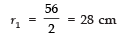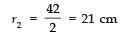Height h = 15 cm
Since the volume of a frustum of a cone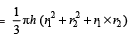∴ Volume of the bucket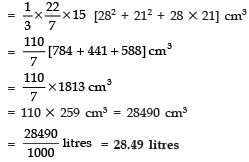Q2. The height of a cone is 30 cm. A small cone is cut off at the top by a plane parallel to the base. If its volume be 1/27th of the volume of the given cone, at what height above the base is the section made?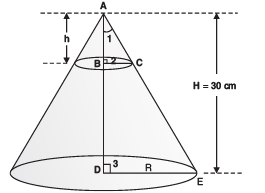Sol. In the figure, we have ΔABC ~ ΔADE

[By AA similarity]

⇒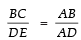[∵ corresponding sides of similar Δs are proportional.]

⇒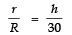...(1)
Here, volume of the small cone =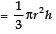Volume of the given cone =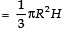Since, [Volume of the small cone] = 1/27
[Volume of the given cone]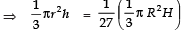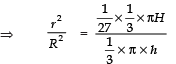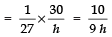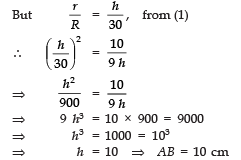∴The required height BD = AD − AB = (30 − 10) cm = 20 cm

Q3. A hollow cone is cut by a plane parallel to the base and the upper portion is removed. If the curved surface of the remainder is 8/9 of the curved surface of the whole cone, find the ratio of the line segments into which the altitude of the cone is divided by the plane.

Sol. In the figure, we have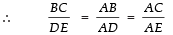[ä sides of similar Δs are proportional.]

⇒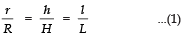Now, curved surface area of the small cone = πrl
Curved surface area of the whole cone = πRL Since,
[Surface area of the remainder (frustum)]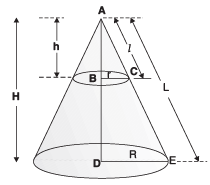=  8/9 [curved surface area of the given cone]

∴ [curved surface area of the frustum]

= 8/9 [curved surface area of the given cone]
⇒ [curved surface area of the small cone]

= 1/9 [curved surface area of the whole cone]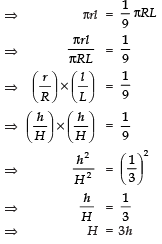Now, BD = H − h = 3h − h = 2h

∴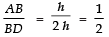⇒ AB : BD = 1 : 2

Q4. A circus tent is cylindrical to a height of 3 m and conical above it. If its base radius is 52.5 m and slant height of the conical portion is 53 m, find the area of the canvas needed to make the tent.

[Use π = 22/7]

Sol. For cylindrical part:

We have, radius (r) = 52.5 m
Height (h)=3 m
Curved surface area = 2πrh
For the conical part
Slant height (l) = 53 m
∴Curved surface area = πrl
Area of the canvas = 2πrh + πrl
= πr (2h + l)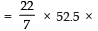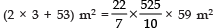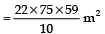= 11 × 15 × 59 m2 =  9735 m2

Q5. Find the number of coins of 1.5 cm diameter and 0.2 cm thickness to be melted to form a right circular cylinder of height 10 cm and diameter 4.5 cm.

Sol. The coin is in a cylindrical form,
∴ Volume of the coin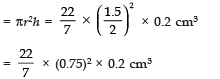Let the number of coins= n
∴Total volume of all coins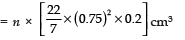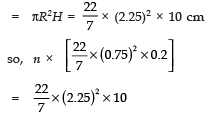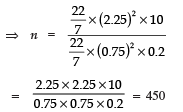Q6. An iron pillar has some part in the form of a right circular cylinder and remaining in the form of a right circular cone. The radius of the base of each of cone and cylinder is 8 cm. The cylindrical part is 240 cm high and the conical part in 36 cm high. Find the weight of the pillar if one cu. cm of iron weighs 7.8 grams.

Sol. Here, height of the cylindrical part h = 240 cm
Height of the conical part, H = 36 cm
Now, the total volume of the pillar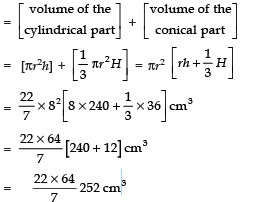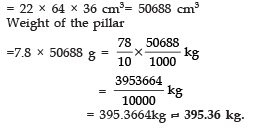Q7. An open container made up of a metal sheet is in the form of a frustum of a cone of height 8 cm with radii of its lower and upper ends as 4 cm and 10 cm respectively. Find the cost of oil which can completely fill the container at the rate of Rs 50 per litre. Also, find the cost of metal used, if it costs Rs 5 per 100 cm2.

[Use π = 3.14]

Sol. Here, for the frustum,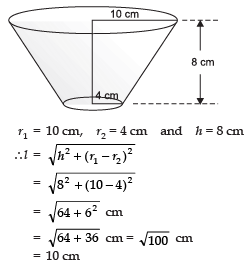∴ Volume of the container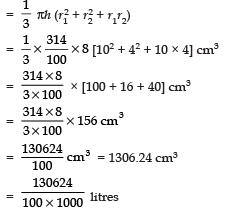= 1.30624 litres ≈ 1.31 approx.

Cost of oil = Rs (1.31 × 50) = Rs 65.50

Now, the total surface area of the container (excluding upper base)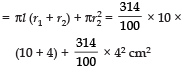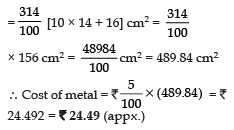Q8. The perimeters of the ends of frustum of a cone are 207.24 cm and 169.56 cm. If the height of the frustum be 8 cm , find the whole surface area of the frustum. [Use π = 3.14]

Sol. Let the radii of circular ends be R and r [ R > r]
Perimeter of circular ends are 207.24 cm and 169.56 cm
∴ 2 π R = 207.24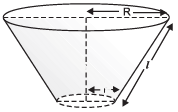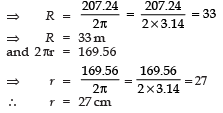Slant-height of the frustum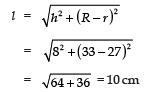The whole surface area of the frustum = π [(R2 + r2) + (R + r)l]
∵ Required whole surface area of the frustum

= 3.14 [332 + 272 +
(33 + 27) × 10] cm2
= 3.14 [1089+ 729 + 600] cm2
= 3.14  cm2  =  7592.52 cm2

Q9. A building is in the form of a cylinder surmounted by a hemispherical dome as shown in the figure. The base diameter of the dome is equal to 2/3 of the total height of the building. Find the height of the building, if it contains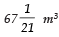of air.

Sol. Here, radius of the hemispherical part = r (say)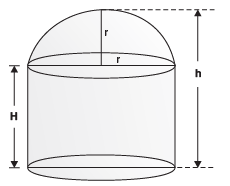Let the total height of the building = h
And the height of the cylindrical part = H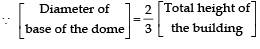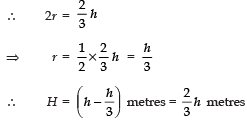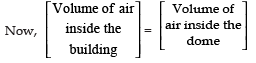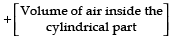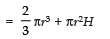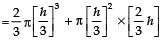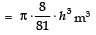But, volume of the air in the building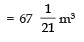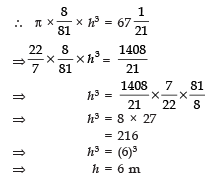Thus, the required height of the building is 6 metres.

Q10. A solid toy is in the form of a hemisphere surmounted by a right circular cone. The height of the cone is 4 cm and diameter of the base is 8 cm. Determine the volume of the toy. If the cube circumscribes the toy, then find the difference of the volumes of the cube and the toy. Also, find the total surface area of the toy.

Sol. Let the radius of the hemisphere = r
And height of the cone = h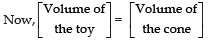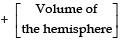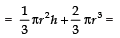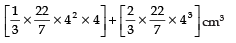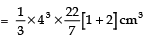Since, the cube circumscribing the given solid must have its edge as (4 + 4) cm i.e., 8 cm,
∴ Volume of the cube =(edge)3 = (8)3 cm3 = 512 cm3
Now, difference in volumes of the cube and the toy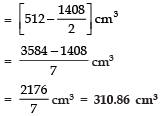Also, total surface area of the toy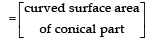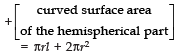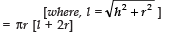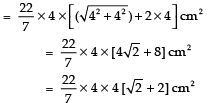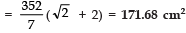Offer running on EduRev: Apply code STAYHOME200 to get INR 200 off on our premium plan EduRev Infinity!

,

,

,

,

,

,

,

,

,

,

,

,

,

,

,

,

,

,

,

,

,

;